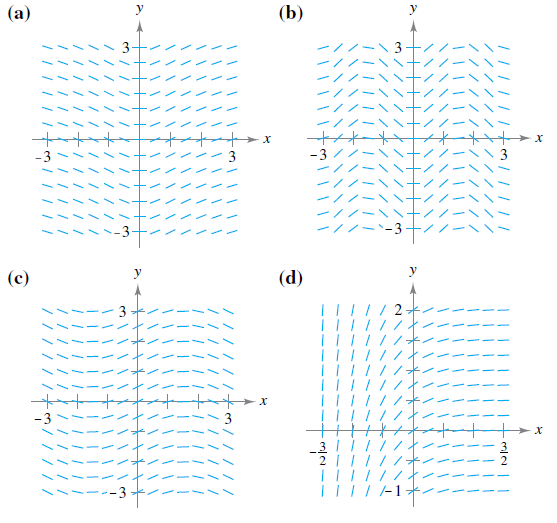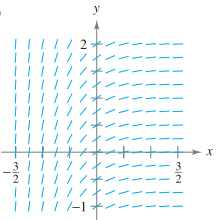Chapter 6.1, Problem 59E

Chapter
Section
Textbook Problem

Matching In Exercises 57–60, match the differential equation with its slope field. [The slope fields are labeled (a), (b), (c), and (d).]d y d x = e − 2 x

To determine

the differential equation associated with its given slope field.Explanation

Given: dydx=e2x and the four graphs of the slope field.

a). b).

c). d).

As dydx=e2x, it implies that the slope is exponential in nature.

We start by creating a table showing the slopes at several points.

The table shown below is a smallsample. The slopes at many other points should be calculated to get a representative slope field

Still sussing out bartleby?

Check out a sample textbook solution.

See a sample solution

The Solution to Your Study Problems

Bartleby provides explanations to thousands of textbook problems written by our experts, many with advanced degrees!

Get Started